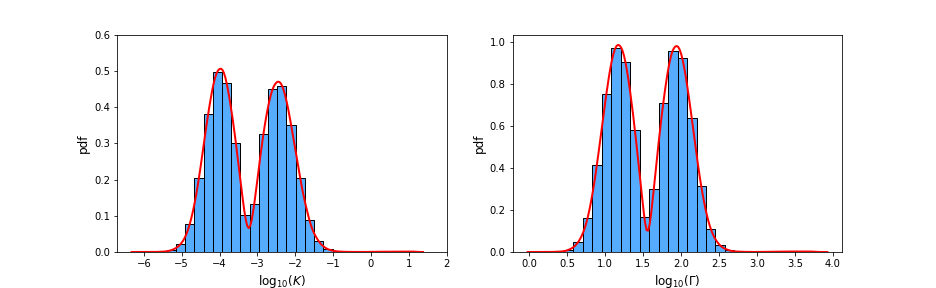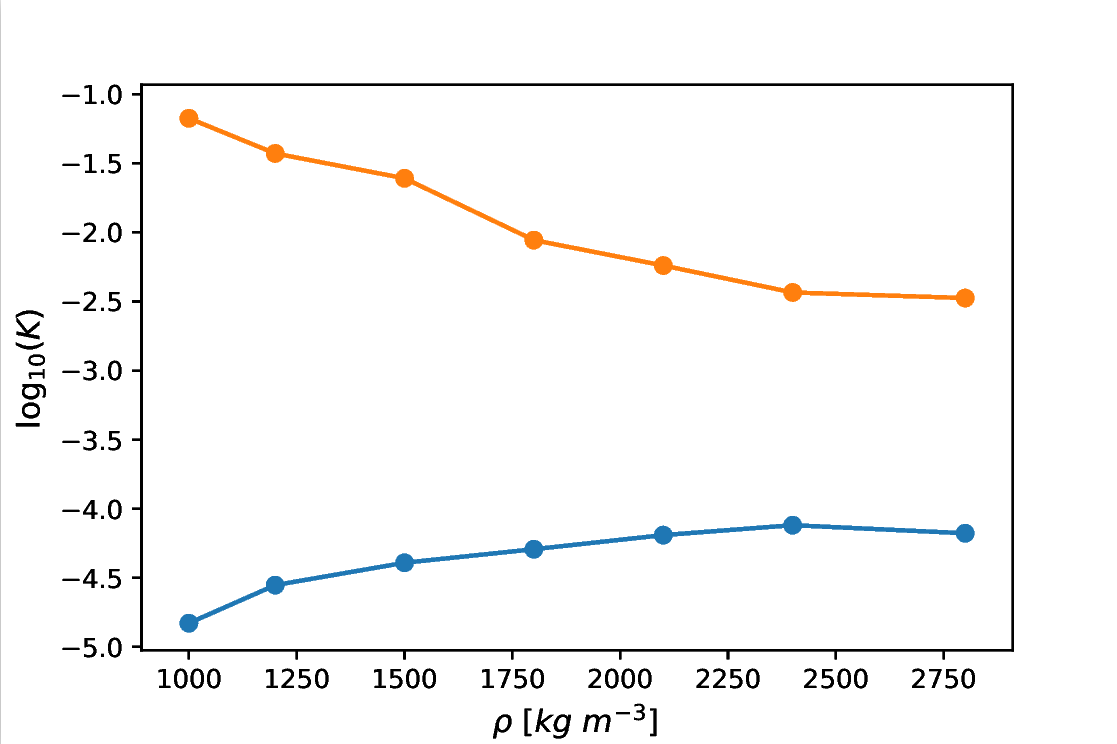Europlanet Science Congress 2021
Virtual meeting
13 September – 24 September 2021
EPSC Abstracts
Vol. 15, EPSC2021-390, 2021, updated on 21 Jul 2021
https://doi.org/10.5194/epsc2021-390
European Planetary Science Congress 2021

# The extended Hayabusa2 mission target 1998 KY26: another small super-fast rotator with low thermal inertia.

Vanja Petković et al.

Introduction:

Surface and internal properties of asteroids are crucial for modeling planetesimal formation, granular mechanics, impact cratering, and thermal evolution of their parent bodies. Additionally, they are essential for designing landing and sample return missions, as well as for a possible deflection scenario. Measurements of the Yarkovsky effect can be used to constrain thermal properties. Recently,  used this approach to estimate the thermal inertia of the small and super-fast rotator 2011 PT, and found it to be unexpectedly low.

In this work we studied the near-Earth object (NEO) 1998 KY26, target of the extended Hayabusa2 mission . This asteroid shares some similar properties with 2011 PT, such as the diameter and the rotation period. We estimated the surface thermal inertia, and then investigated the possibility for it to be a rubble-pile or have a surface regolith layer. Further characterization of this object would be useful not only for the Hayabusa2 mission, but also for studying other NEOs.

Methods:

Thermal properties: Surface thermal conductivity has been estimated using the probabilistic model developed in . This model is based on the comparison between the Yarkovsky effect predicted by an analytical model , and the measurements obtained from astrometry. The analytical model depends on several parameters: the semi-major axis of the orbit, the diameter of the object, the density, the thermal conductivity, the heat capacity, the obliquity, and the rotation period. The thermal conductivity K is unknown, while all the other parameters are either measured, or modeled using a suitable distribution. Assuming that a measurement of the Yarkovsky effect is available, we can solve the model vs observed Yarkovsky drift equation and determine a distribution of K using a Monte Carlo method.

The semi-major axis of 1998 KY26 is a = 1.2328 au, with a very small uncertainty, so we kept it fixed. Radar observations  suggest a diameter between 20 and 40 meters, hence we modeled this parameter using a Gaussian distribution with μ = 30 m and σ = 6 m. For the density ρ we used a lognormal distribution with mean value 2800 kg m−3 , and a standard deviation of 400 kg m−3. To understand how the result depends on ρ, we also tested lognormal distributions with mean equal to 1000, 1200, 1500, 1800, 2100, and 2400 kg m−3 and the aforementioned standard deviation. The heat capacity C is unknown, and we fixed it at 800 J kg−1 K−1. The obliquity is also unknown, and we used a population-based distribution . The rotation period has been measured to be P = 10.7015 ± 0.0004 min . The semi-major axis drift reported in the JPL Small Body Database is da/dt = -0.0116 ± 0.002 au My−1. The errors of these two parameters were modeled using a Gaussian distribution.

Critical spin period and regolith preservation: We used an analytical model  to compute the critical spin period Tc for different values of bulk cohesion and density. Additionally, we calculated its mean normal stress p to see if this asteroid is in the tensile or compressive regime. Finally, we implemented a model  for studying the stability of surface regolith and its preservation.

Results:

We found that the probability density function (PDF) for K is always bimodal with very low values (Fig. 1). For the nominal value of the density ρ = 2800 kg m−3 the most likely values of K are 7·10-5 and 3·10-3 W m-1 K-1. The corresponding values of thermal inertia Γ are 12 and 67 J m-2 K-1 s-1/2. Fig. 2 shows how the peaks depend on the density, and we can notice that they approach each other as ρ increases.Fig. 1 PDF of K (left) and Γ (right) for ρ = 2800 kg m−3 and  C = 800 J kg-1 K-1

For bulk cohesion values of several pascals Tc remains lower than P. The resulting value of p = 8.5 Pa suggests that the asteroid is in a tensile regime for any given value of ρ. We found that the minimum cohesion needed to keep 1 mm regolith particles on the whole surface is of the order of 10−3 Pa.Fig. 2 Thermal conductivity maxima for different density distributions (C = 800 J kg−1 K−1 ).

Summary and conclusions:

The thermal conductivity values estimated for 1998 KY26 are unexpectedly low for small fast rotators. Therefore, the asteroid either has a regolith layer on its surface or a high porosity that might be related to a rubble-pile structure. The model we applied for the stability of a surface regolith layer on a supposed monolithic object shows that even a weak cohesion strength may permit small particles to stay at the surface. On the other hand, even if the asteroid has a rubble-pile internal structure, we show that it can still survive the fast rotation when the cohesion is above a few pascals. The required cohesion agrees with the available data for small solar system objects.

References:

 Fenucci, M. et al. (2021), A&A, 647, A61

 Hirabayashi, M. et al. (2021), Adv. Space. Res., in press

 Vokrouhlický, D. (1999), A&A, 344, 362-366

 Ostro, S. J. et al. (1999), Science, 285, 557-559

 Tardioli, C. et al. (2017), A&A, 608, A61

 Hu, S. et al. (2021) , MNRAS, 502, 5277

 Li, X. & Scheeres, D. J. (2021), Icarus, 357, 114249

How to cite: Petković, V., Fenucci, M., and Novaković, B.: The extended Hayabusa2 mission target 1998 KY26: another small super-fast rotator with low thermal inertia., European Planetary Science Congress 2021, online, 13–24 Sep 2021, EPSC2021-390, https://doi.org/10.5194/epsc2021-390, 2021.